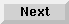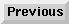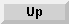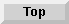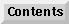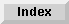3.4 Using optimized constructs

# 3.4.1 Tail call optimization

Unless directed otherwise, the Compiler optimizes self-recursive function calls, tail calls, and self-tail calls. In particular, self-tail calls are automatically compiled as loops. While these function calls are efficient, they can be difficult to trace because they do not appear on the stack.

• A function call is a self-recursive call if the function calls itself. For example, the following code contains a recursive function call to`fact`:

```(defun fact (n)
(if (< n 1) 1 (* n (fact (1- n)))))

```
Unless compiled as`notinline`, self-recursive function calls jump directly to the start of the code rather than going through the`symbol-function` slot. A trace of a self-recursive function shows only the initial call, not the resulting recursive calls. For example, if you trace`fact`, a call to (`fact2`) shows only the initial call to`fact`, not the recursive calls to (`fact1`) and (`fact0`).

• A function call is a tail call if it is the last operation performed by the calling function. In the following code, all of the function calls in the case statement are tail calls:

```(defun analyze-cereal (cereal)
(case (cereal-kind cereal)
(oat (analyze-oat-cereal cereal))
(wheat (analyze-wheat-cereal cereal))
(sugar (analyze-sugar-cereal cereal))
(t (analyze-other-cereal cereal))))

```
• A function call is a self-tail call if it calls itself as the last operation. In the following example, the call to`my-last` is a self-tail call:

```(defun my-last (x)
(if (cdr x) (my-last (cdr x)) x))

```
A function defined using self-tail calls is a tail-recursive function. Because of tail merging, tail-recursive functions are usually as efficient as functions defined using iterative constructs.

When the Compiler compiles either a tail call or a self-tail call, it reuses the calling function's stack frame rather than creating a new stack frame. This optimization is called tail merging.

The Advanced User's Guide - 9 SEP 1996Generated with Harlequin WebMaker